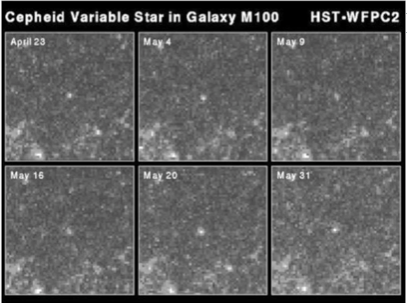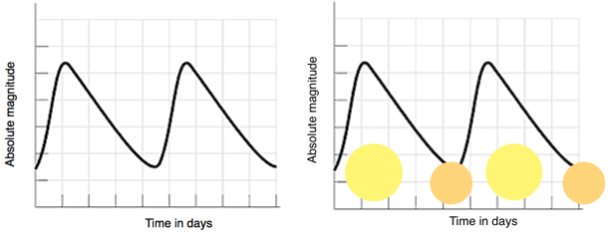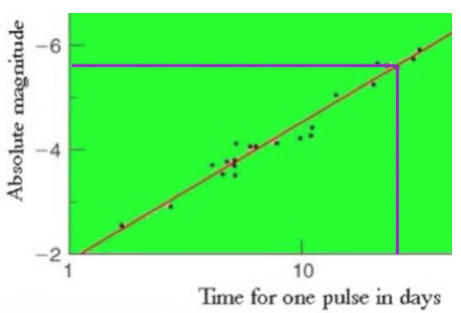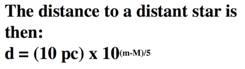### Cepheid variable stars

A Cepheid variable star is pictured in the centre of these frames from NASA taken over a period of time. The stars pulse regularly, their brightness changing by a large amount over periods of days.Nasa photo of Pulsing Cepheid variable

The usually accepted explanation for the pulsing effect involves helium in the stars atmosphere.. Doubly ionised helium (where both electrons have been stripped off) is more opaque than singly ionized helium. The more helium is heated, the more ionised it becomes. At the dimmest part of a Cepheid's cycle, the ionised gas in the outer layers of the star is opaque, and so is heated by the star's radiation, and due to the increased temperature, begins to expand. As it expands, it cools, and so becomes less ionised and therefore more transparent, allowing the radiation to escape. Then the expansion stops, and reverses due to the star's gravitational attraction.

The whole process then repeats itself at very regular predictable intervals.Cepheid variable pulse rate graph

The astronomer Henrietta Swan Leavitt discovered that there was a connection between the pulse rate of the Cepheids and the brightness or absolute magnitude. This discovery enabled these stars to be used for distance measurement. In particular Edwin Hubble used the idea to estimate the distances of galaxies containing Cepheids in his formulation of “Hubble’s Law”.Absolute magnitude from graph of pulse rate

From the pulse rate we use the graph to estimate the absolute magnitude of the star. We then calculate the distance comparing the apparent magnitude with the absolute magnitude.Absolute magnitude to distance calculation M is the absolute magnitude and m the apparent magnitude

The video below explains the estimate of distance using Hertzsprung Russell diagrams and Cepheid variables